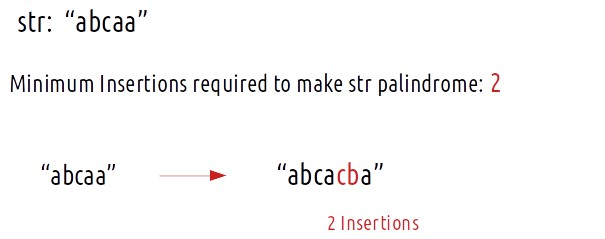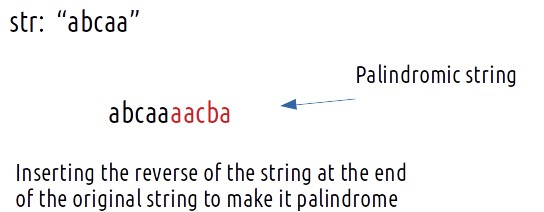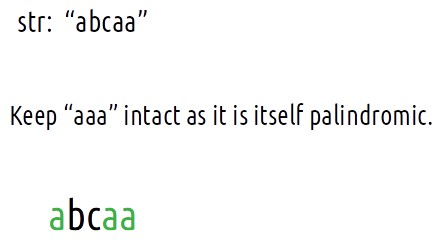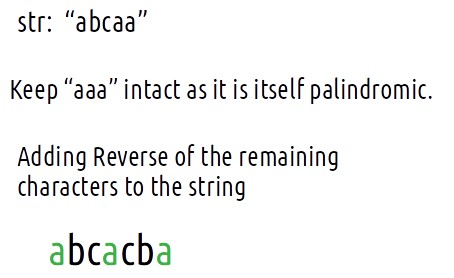# Minimum insertions to make string palindrome | DP-29

Problem Statement: Minimum insertions required to make a string palindrome

A palindromic string is a string that is the same as its reverse. For example: “nitin” is a palindromic string. Now the question states that we are given a string, we need to find the minimum insertions that we can make in that string to make it a palindrome.

```Example:```

Problem Link: Minimum Insertions to make a string palindrome

### Intuition:

We need to find the minimum insertions required to make a string palindrome. Let us keep the “minimum” criteria aside and think, how can we make any given string palindrome by inserting characters?

The easiest way is to add the reverse of the string at the back of the original string as shown below. This will make any string palindrome.Here the number of characters inserted will be equal to n (length of the string). This is the maximum number of characters we can insert to make strings palindrome.

The problem states us to find the minimum of insertions. Let us try to figure it out:

• To minimize the insertions, we will first try to refrain from adding those characters again which are already making the given string palindrome. For the given example, “aaa”, “aba”,”aca”, any of these are themselves palindromic components of the string. We can take any of them( as all are of equal length) and keep them intact. (let’s say “aaa”).• Now, there are two characters(‘b’ and ‘c’) remaining which prevent the string from being a palindrome. We can reverse their order and add them to the string to make the entire string palindrome.We can do this by taking some other components (like “aca”) as well.

In order to minimize the insertions, we need to find the length of the longest palindromic component or in other words, the longest palindromic subsequence.

Minimum Insertion required = n(length of the string) – length of longest palindromic subsequence.

Approach:

The algorithm is stated as follows:

• We are given a string (say s), store its length as n.
• Find the length of the longest palindromic subsequence ( say k) as discussed in dp – 28
• Return n-k as answer.

Code:

## C++ Code

``````#include <bits/stdc++.h>

using namespace std;

int lcs(string s1, string s2) {

int n=s1.size();
int m=s2.size();

vector<vector<int>> dp(n+1,vector<int>(m+1,-1));
for(int i=0;i<=n;i++){
dp[i] = 0;
}
for(int i=0;i<=m;i++){
dp[i] = 0;
}

for(int ind1=1;ind1<=n;ind1++){
for(int ind2=1;ind2<=m;ind2++){
if(s1[ind1-1]==s2[ind2-1])
dp[ind1][ind2] = 1 + dp[ind1-1][ind2-1];
else
dp[ind1][ind2] = 0 + max(dp[ind1-1][ind2],dp[ind1][ind2-1]);
}
}

return dp[n][m];
}

int longestPalindromeSubsequence(string s){
string t = s;
reverse(s.begin(),s.end());
return lcs(s,t);
}

int minInsertion(string s){
int n = s.size();
int k = longestPalindromeSubsequence(s);
return n-k;
}

int main() {

string s= "abcaa";
cout<<"The Minimum insertions required to make string palindrome: "<<
minInsertion(s);
}
``````

Output:

The Minimum insertions required to make string palindrome: 2

Time Complexity: O(N*N)

Reason: There are two nested loops

Space Complexity: O(N*N)

Reason: We are using an external array of size (N*N). Stack Space is eliminated.

## Java Code

``````import java.util.*;

class TUF{
static int lcs(String s1, String s2) {

int n=s1.length();
int m=s2.length();

int dp[][]=new int[n+1][m+1];
for(int rows[]:dp)
Arrays.fill(rows,-1);
for(int i=0;i<=n;i++){
dp[i] = 0;
}
for(int i=0;i<=m;i++){
dp[i] = 0;
}

for(int ind1=1;ind1<=n;ind1++){
for(int ind2=1;ind2<=m;ind2++){
if(s1.charAt(ind1-1)==s2.charAt(ind2-1))
dp[ind1][ind2] = 1 + dp[ind1-1][ind2-1];
else
dp[ind1][ind2] = 0 + Math.max(dp[ind1-1][ind2],dp[ind1][ind2-1]);
}
}

return dp[n][m];
}

static int longestPalindromeSubsequence(String s){
String t = s;
String ss=new StringBuilder(s).reverse().toString();
return lcs(ss,t);
}

static int minInsertion(String s){
int n = s.length();
int k = longestPalindromeSubsequence(s);
return n-k;
}

public static void main(String args[]) {

String s= "abcaa";
System.out.println("The Minimum insertions required to make string palindrome: "+
minInsertion(s));
}
}``````

Output:

The Minimum insertions required to make string palindrome: 2

Time Complexity: O(N*N)

Reason: There are two nested loops

Space Complexity: O(N*N)

Reason: We are using an external array of size (N*N). Stack Space is eliminated.

### Space Optimization

If we closely we are using two rows: dp[ind1-1][ ], dp[ind][ ],

So we are not required to contain an entire array, we can simply have two rows prev and cur where prev corresponds to dp[ind-1] and cur to dp[ind].

After declaring prev and cur, replace dp[ind-1] to prev and dp[ind] with cur and after the inner loop executes, we will set prev = cur, so that the cur row can serve as prev for the next index.

Code:

## C++ Code

``````#include <bits/stdc++.h>

using namespace std;
int lcs(string s1, string s2) {

int n=s1.size();
int m=s2.size();

vector<int> prev(m+1,0), cur(m+1,0);

// Base Case is covered as we have initialized the prev and cur to 0.

for(int ind1=1;ind1<=n;ind1++){
for(int ind2=1;ind2<=m;ind2++){
if(s1[ind1-1]==s2[ind2-1])
cur[ind2] = 1 + prev[ind2-1];
else
cur[ind2] = 0 + max(prev[ind2],cur[ind2-1]);
}
prev= cur;
}

return prev[m];
}

int longestPalindromeSubsequence(string s){
string t = s;
reverse(s.begin(),s.end());
return lcs(s,t);
}

int minInsertion(string s){
int n = s.size();
int k = longestPalindromeSubsequence(s);
return n-k;
}

int main() {

string s= "abcaa";

cout<<"The Minimum insertions required to make string palindrome: "<<
minInsertion(s);
}
``````

Output:

The Minimum insertions required to make string palindrome: 2

Time Complexity: O(N*M)

Reason: There are two nested loops.

Space Complexity: O(M)

Reason: We are using an external array of size ‘M+1’ to store only two rows.

## Java Code

``````import java.util.*;

class TUF{
static int lcs(String s1, String s2) {

int n=s1.length();
int m=s2.length();

int[] prev=new int[m+1];
int[] cur=new int[m+1];

// Base Case is covered as we have initialized the prev and cur to 0.

for(int ind1=1;ind1<=n;ind1++){
for(int ind2=1;ind2<=m;ind2++){
if(s1.charAt(ind1-1)==s2.charAt(ind2-1))
cur[ind2] = 1 + prev[ind2-1];
else
cur[ind2] = 0 + Math.max(prev[ind2],cur[ind2-1]);
}

prev = (int[])(cur.clone());

}

return prev[m];
}

static int longestPalindromeSubsequence(String s){
String t = s;
String ss=new StringBuilder(s).reverse().toString();
return lcs(ss,t);
}

static int minInsertion(String s){
int n = s.length();
int k = longestPalindromeSubsequence(s);
return n-k;
}

public static void main(String args[]) {

String s= "abcaa";
System.out.println("The Minimum insertions required to make string palindrome: "
+minInsertion(s));
}
}``````

Output:

The Minimum insertions required to make string palindrome: 2

Time Complexity: O(N*M)

Reason: There are two nested loops.

Space Complexity: O(M)

Reason: We are using an external array of size ‘M+1’ to store only two rows.

Special thanks to Anshuman Sharma for contributing to this article on takeUforward. If you also wish to share your knowledge with the takeUforward fam, please check out this article